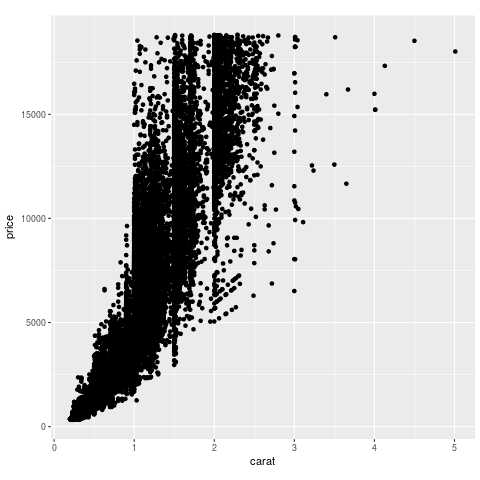In :
:ext QuasiQuotes
import qualified H.Prelude as H
H.initialize H.defaultConfig


## Doing things in R¶

In :
-- print output
[rprint| lm(mpg ~ wt, data = mtcars) |]

Call:
lm(formula = mpg ~ wt, data = mtcars)

Coefficients:
(Intercept)           wt
37.285       -5.344
In :
-- plot stuff
-- we just require ggplot2 as we do everything directly in R
[rgraph|
require(ggplot2)
ggplot(diamonds, aes(carat, price)) + geom_point() + theme(aspect.ratio = 1) |]Assignment also works as usual:

In :
[rprint| fact <- function(n) if(n == 0) 1 else n * fact(n - 1)
fact(10) |]

 3628800

Call fact again...

In :
[rprint| fact(10) |]

R Runtime Error: Error in fact(10) : could not find function "fact"

The embedded R interpreter is stateful, but for global assignment we need to use <<-

In :
[r| fact <<- function(n) if(n == 0) 1 else n * fact(n - 1) |]

0x00007f86bf8df678
In :
[rprint| fact(25) |]

 1.551121e+25

## Fine, but my data are in Haskell ... how do I ship them over to R?¶

The _hs suffix is used to splice values from Haskell into the R code:

In :
-- a Haskell list
xs = [1..10] :: [Double]
xs

[1.0,2.0,3.0,4.0,5.0,6.0,7.0,8.0,9.0,10.0]
In :
[rprint| mean(xs_hs) |]

 5.5

We can even use functions defined in Haskell in R:

In :
import Data.Int
fact :: Int32 -> Int32
fact 0 = 1
fact n = n * fact (n - 1)

factR x = return $fact x :: H.R s Int32  In : n = 6 :: Int32 [rprint| factR_hs(n_hs) |]   720 ## What if I want to use results from R computations in Haskell?¶ In : get_normals :: Double -> H.R s [Double] get_normals n = do H.dynSEXP <$> [r| rnorm(n_hs) |]
result <- H.runRegion \$ get_normals 4

result

[1.1294181047596081,0.1277917949807154,-0.11111631846148341,1.878786090403989]

Now these are just normal Haskell values you can work with:

In :
:t result

result :: [Double]